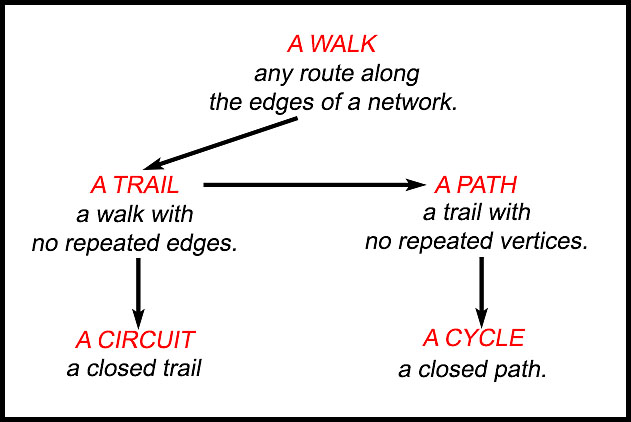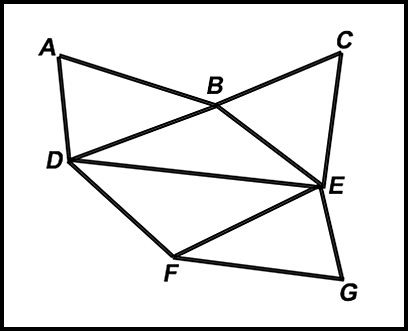Dr. J's Maths.com
Where the techniques of Maths
are explained in simple terms.

Networks - Walks, paths and cycles.
Test Yourself 1.

The following path is a directed (but unweighted) network showing a minimum spanning tree to help you remember the basic definitions:Walks 1. Paths 7. Cycles 13. Mixed questions. 19.In the above diagram, describe the path indicated in terms of the above five descriptions: (i) ABCEBA walk repeats vertices B and A as well as edge AB (ii) ABCEDA trail (has a repeated vertex A (iii) DBEFG path no repeated vertices (iv) DEGFD cycle no repeated edges